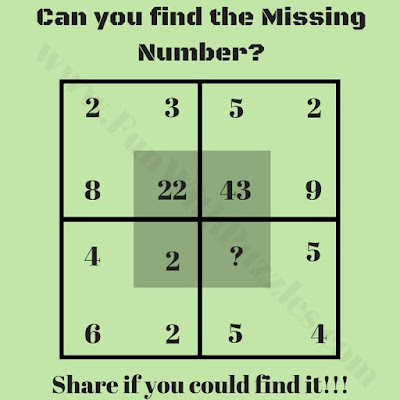## Monday, May 21, 2018

This Brain Maths Question is for school going kids. In this Maths Puzzle Question, there are some numbers given in four squares. Numbers in each of the square relates to each other Mathematically. Can you decipher this Mathematical relationship or pattern among the numbers in each of the square and then find the missing number which will replace the question mark in one of the square?Can you find the missing number?

Answer of this "Brain Maths Question for Students", is hidden as of now to avoid any spoiler. Do post your answers in the comments box. Also you can post your request to view the answer in the comment section. Please do give your best try to solve this brain teaser.

1.1.